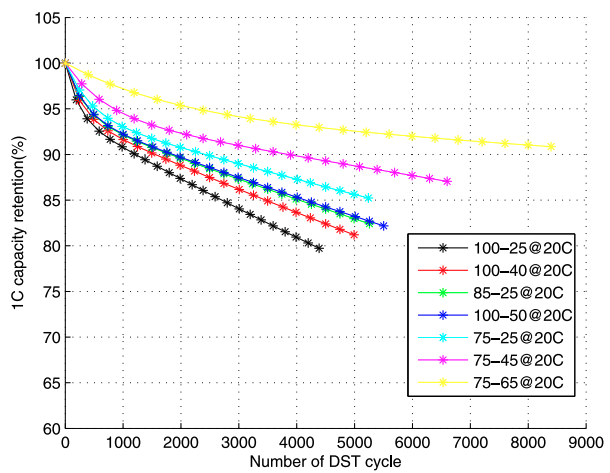The model predicts battery capacity degradation based on state-of-charge profile and cell temperature, reference here.

Code in Matlab.

#### Piece-wise linear degradation model for optimization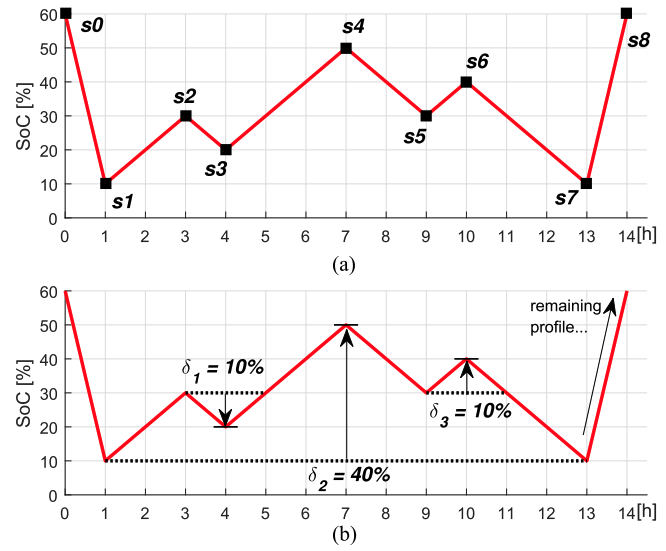This is a piece-wise linear model for incorporating nonlinear battery cycle degradation mechanisms into optimization, reference here.

Code in GAMS (requires Gurobi).

#### Optimal battery control and bidding in frequency regulationThis code demonstrates the optimal response control and bidding design for degradation-sensitive batteries in PJM pay-for-performance frequency regulation markets, reference here.

Code in Matlab.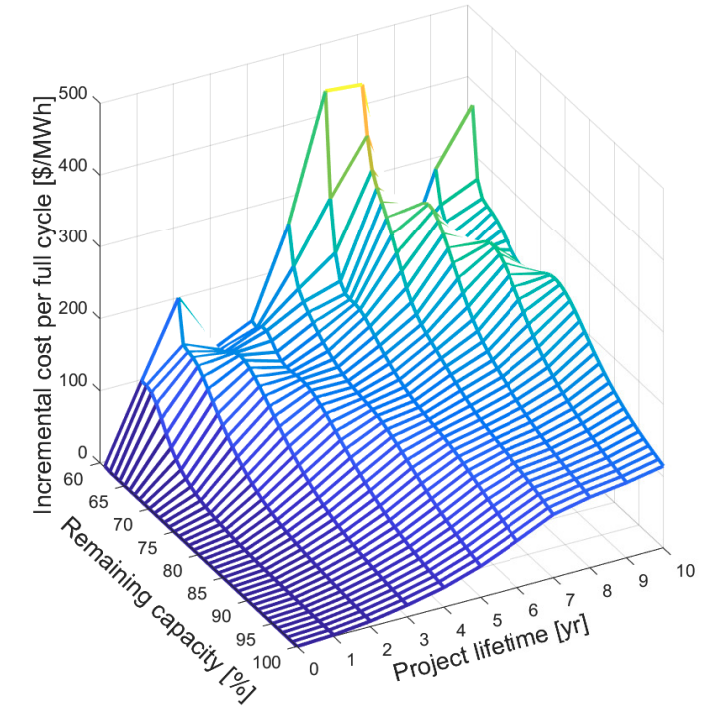This code calculates the opportunity value of battery degradation based on historical price data using dynamic programming, reference here.

Code in Julia (requires Gurobi).

#### Battery price arbitrage using stochastic dynamic programming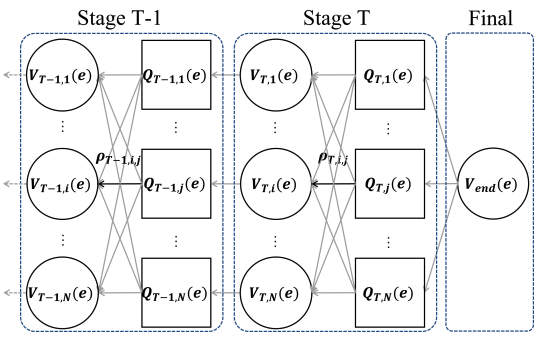The code uses an analytical algorithm to solve stochastic dynamic programming for optimizing battery price arbitrage in wholesale real-time electricity markets, reference here.

Code in Matlab.

#### Comparing different battery degradation models in power system optimizationThe code compares different degradation models in grid optimization. Reference paper is here.

Code in Julia (requires Gurobi).

#### Transferable energy storage bidderAlgorithms for bidding energy storage to arbitrage in wholesale markets. Reference paper is here.

Code in Python.

#### Assessing market participation for energy storage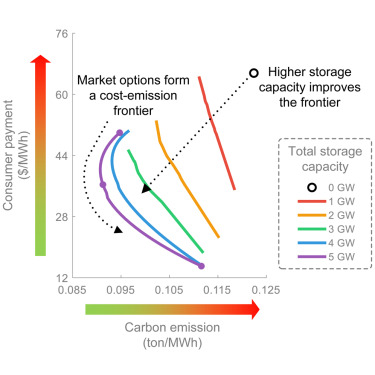A two-stage market clearing simulation framework to assess energy storage participation in day-ahead and real-time markets. Reference paper is here.

Code in Matlab.